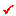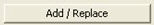# Section Stiffness Scale Factor

Apply scale factors to the cross-sectional properties of linear elements (Truss, Tension-only, Compression-only, Cable, Gap, Hook & Beam Elements). Specific stiffness of specific member types may be reduced such as the case where the flexural stiffness of lintel beams and walls may require reduction to reflect cracked sections of concrete.

This function becomes a convenient tool for reducing flexural stiffness by member types.  Also, section stiffness scale factors can be included in boundary groups, which can be applied to individual construction stages in a construction stage analysis.  The scale factors are also applied to composite sections for construction stages.  When stiffness scale factors are defined for a composite section for construction stages (Load > Construction Stage Analysis Data > Composite Section for Construction Stage), the stiffness scale factors for each Section Part are applied together with the section stiffness scale factors defined here.

The scale factors defined here are applied to the calculations for displacements and member forces only.  For stress calculations, original section properties are used.  However, the option for applying the Section Stiffness Scale Factors to Stress Calculations may be selected in Analysis > Main Control Data.

"Model>Boundary>Effective Width Scale Factor" is similar to "Section Stiffness Scale Factor" and is used when considering the effective width of a section.

The scale factors applied in "Effective Width Scale Factor" are used for stress calculations only, and they are treated as boundary conditions so that the stiffness reflecting the effective width can be used in a specific construction stage.

The scale factors defined in "Section Stiffness Scale Factor" are saved as section data and used for each construction stage as well as the final stage.

From the Main Menu select Model > Properties > Section Stiffness Scale Factor.

## Section Scale Factor dialog box

####Boundary Group Name

Select a boundary group in which Section Stiffness Scale Factors will be included.  This function is used when we wish to change boundary conditions with different section properties for different load cases.   In order to change section properties with different boundary conditions, enter different Section Stiffness Scale Factor for each boundary condition group.

In case section properties need to be changed in Construction Stage Analysis, the Section Stiffness Scale Factor corresponding to the boundary group is applied by activating the boundary group at the corresponding construction stage. Note that the applied Section Stiffness Scale Factor will remain unchanged even after the corresponding boundary group is deactivated. For example, if we wish to apply a scale factor of 0.5 at  CS1 and subsequently change to 1.0 at CS2, the scale factor of 0.5 remains unchanged even after deactivating the boundary group corresponding to the scale factor of 0.5. To achieve what is intended, we need to define a boundary group pertaining to the scale factor of 1.0 and activate the boundary group at CS2.

####Section

Select the sections that require stiffness changes from the list, which lists all the input sections.

####Scale Factor

Enter the required scale factors to be applied to each stiffness component.

I = J  : Applicable only for tapered sections. Section Stiffness Scale Factors at I-end and J-end can be different.

Area: Cross-sectional area

Asy: Effective Shear Area resisting shear forces in the element local y-axis

Asz: Effective Shear Area resisting shear forces in the element local z-axis

Ixx: Torsional Resistance about the element local x-axis

Iyy: Area Moment of Inertia about the element local y-axis

Izz: Area Moment of Inertia about the element local z-axis

Weight: Cross-sectional area used for calculating self-weight: Add or modify reduced stiffness data of each member type in a spreadsheet format table.

Note
Copy & Paste and Edit the data in MS-Excel.Composite Section

For sections defined as Composite Type, Scale Factors can be defined for Before (before composite action), After (after composite action) and All.

Before: In case of a composite section, the defined Scale Factor is used to calculate section forces and stresses before composite action.

After: In case of a composite section, the defined Scale Factor is used to calculate section forces and stresses after composite action.

All: In case All (Before and After) is checked on, the defined Scale Factor is used to calculate section forces and stresses before and after composite action.

Note
When a ratio of Modulii of Elasticity for long term and shrinkage is defined in Section Data, the structure group for long term and shrinkage is automatically generated, and the applied scale factors for each are displayed.